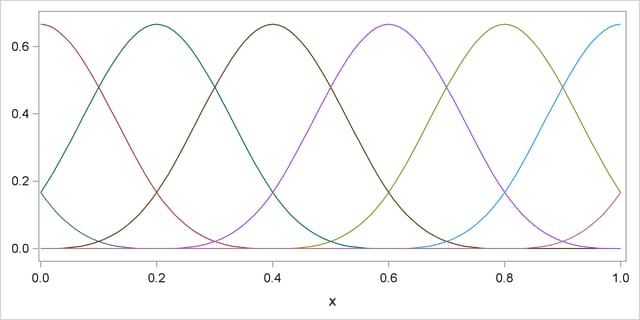### B-Spline Basis

A B-spline basis can be built by starting with a set of Haar basis functions, which are functions that are 1 between adjacent knots and 0 elsewhere, and then applying a simple linear recursion relationship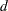times, yielding the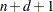needed basis functions. For the purpose of building the B-spline basis, theprespecified knots are referred to as internal knots. This construction requiresadditional knots, known as boundary knots, to be positioned to the left of the internal knots, and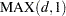boundary knots to be positioned to the right of the internal knots. The actual values of these boundary knots can be arbitrary. The EFFECT statement provides several methods for placing the needed boundary knots, including the common method of using repeated values of the data extremes as the boundary knots. The boundary knot placement affects the precise form of the basis functions that are generated, but it does not affect the following two desirable properties:

1. The B-spline basis functions are nonzero over an interval that spans at most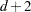knots. This yields design matrix columns each of whose rows contain at mostadjacent nonzero entries.

2. The computation of the basis functions at anyvalue is numerically stable and does not require evaluating powers of this value.

The following figures show the B-spline bases defined on
with four equally spaced internal knots at 0.2, 0.4, 0.6, and 0.8.

Figure 19.2 shows a linear B-spline basis. Note that this basis consists of six functions each of which is nonzero over an interval that spans at most three knots.

Figure 19.2 Linear B-Spline Basis with Four Equally Spaced Interior Knots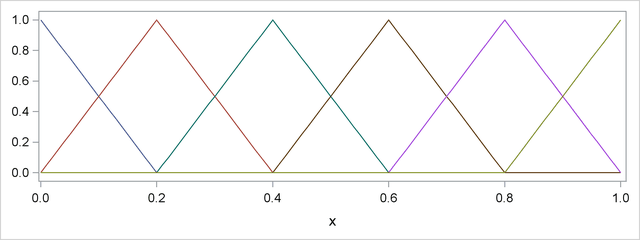Figure 19.3 shows a cubic B-spline basis where the needed boundary knots are positioned at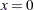and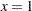. Note that this basis consists of eight functions, each of which is nonzero over an interval spanning at most five knots.

Figure 19.3 Cubic B-Spline Basis with Four Equally Spaced Interior Knots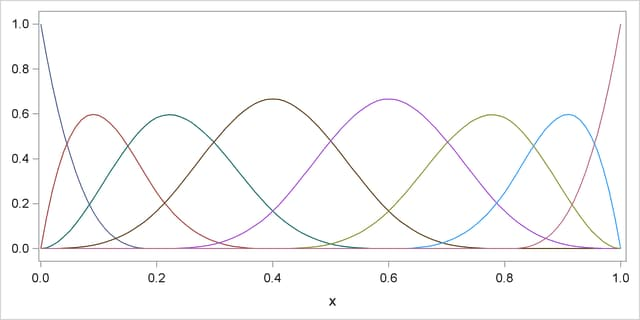Figure 19.4 shows a different cubic B-spline basis where the needed left-side boundary knots are positioned at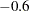,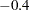,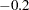, and. The right-side boundary knots are positioned at,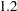,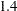, and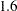. Note that, as in the basis shown in Figure 19.3, this basis consists of eight functions, each of which is nonzero over an interval spanning at most five knots. The different positioning of the boundary knots has merely changed the shape of the individual basis functions.

Figure 19.4 Cubic B-Spline Basis with Equally Spaced Boundary and Interior Knots